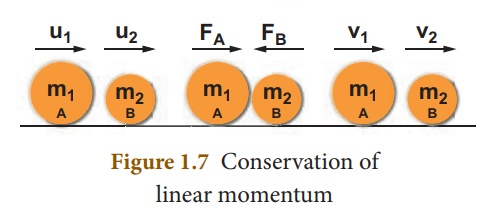Home | | Science 10th Std | Principle of Conservation of Linear Momentum

# Principle of Conservation of Linear Momentum

There is no change in the linear momentum of a system of bodies as long as no net external force acts on them.

PRINCIPLE OF CONSERVATION OF LINEAR MOMENTUM

There is no change in the linear momentum of a system of bodies as long as no net external force acts on them.

Let us prove the law of conservation of linear momentum with the following illustration:## Proof:

Let two bodies A and B having masses m1 and m2 move with initial velocity u1 and u2 in a straight line. Let the velocity of the first body be higher than that of the second body. i.e., u1>u2 . During an interval of time t second, they tend to have a collision. After the impact, both of them move along the same straight line with a velocity v1 and v2 respectively.

Force on body B due to A,

FB= m2 (v2â€“u2)/t

Force on body A due to B,

FA = m1 (v1â€“u1)/t

By Newtonâ€™s III law of motion,

Action force = Reaction force

FA    =         â€“FB

m1 (v1-u1)/t  =       â€“m2 (v2-u2)/t

m1v1 + m2v2 = m1u1 + m2u2 ------ (1.9)

The above equation confirms in the absence of an external force, the algebraic sum of the momentum after collision is numerically equal to the algebraic sum of the momentum before collision.

Hence the law of conservation linear momentum is proved.

Study Material, Lecturing Notes, Assignment, Reference, Wiki description explanation, brief detail
10th Science : Chapter 1 : Laws of Motion : Principle of Conservation of Linear Momentum |